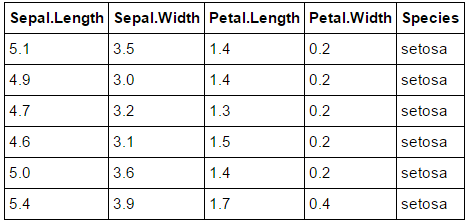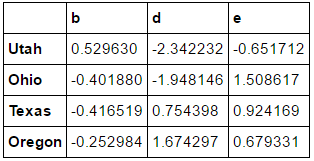# [PYTHON] Is R's do.call () a classical higher-order function? Learn how to use

Looking at Kaggle kernels, which introduces Kaggle's participant code, I saw an R code that makes heavy use of `do.call ()`. Since `do.call ()` was almost new to me, I looked it up and found that it is a relatively classical function and is not difficult to use. Make a note below so that you do not forget it.

## Overview of do.call ()

First, I will quote from the CRAN manual.

do.call - Execute a Function Call

Description

do.call constructs and executes a function call from a name or a function and a list of arguments to be passed to it.

Usage `do.call(what, args, quote = FALSE, envir = parent.frame())`

Arguments

• what either a function or a non-empty character string naming the function to be called.
• args a list of arguments to the function call. The names attribute of args gives the argument names.
• quote a logical value indicating whether to quote the arguments.
• envir an environment within which to evaluate the call. This will be most useful if what is a character string and the arguments are symbols or quoted expressions.

As a function, it is a "Function Call". The R language has a rich set of Apply functions, so it seems that it is famous, but it seems that this do.call () is also used depending on the case. It seems to take four arguments as described above, but the first two are required, the function object "what" and the argument "args" to be passed to it. "args" must be a list variable.

Here are some usage examples.

First, define the function.

``````# define my own function
myrange <- function (larg) {
nv <- unlist(larg)
rg <- max(nv) - min(nv)
return(rg)
}
``````

Here, we use "iris" which can be referred to immediately by R.

``````# Data.Frame example
``````

Table 1. Iris DatasetDo.call () the defined function "myrange".

``````do.call(myrange, list(iris\$Sepal.Length))
# Out: 3.6
``````

As expected, the maximum value of `Sepal.Lengh`-the minimum value (3.6) was output. For the time being, when calculated with the R built-in range (), it was 4.3, 7.9 (minimum value, maximum value), so the solution is in agreement with 3.6 (= 7.9 --4.3) above.

Let's check another example. First, prepare a function that normalizes the numerical value. Prepare the input data sample and execute do.call () as follows.

``````normalize <- function(x, m=mean(x), s=sd(x)) {
(x - m) /s
}

myseq = list(c(1, 3, 6, 10, 15))
do.call(normalize, myseq)

# -1.0690449676497 -0.712696645099798 -0.17817416127495 0.534522483824849 1.4253932901996
``````

The average and standard deviation of the output numerical list are

``````mean of normalized =
 -5.572799e-18
standard deviation =
 1
``````

Since it is a value near 0 and 1 as shown in, it can be seen that the expected normalize can be executed.

## Compare with apply () in Python Pandas

It seems that R's do.call () is similar to the Python built-in function map (), but I don't use it much personally, so this time I will compare it with Pandas' apply (). (Reference: "Python for Data Analysis" --O'reilly media) First, prepare sample data.

``````# Sample Data
frame = pd.DataFrame(np.random.randn(4,3), columns=list('bde'),
index=['Utah', 'Ohio', 'Texas', 'Oregon'])
frame
``````

** Table 2. Data Example**Prepare a function to calculate the range (maximum value-minimum value) and apply () it to pd.DataFrame.

``````# define lambda function
f = lambda x: x.max() - x.min()
frame[['d']].apply(f)
# if I execute frame['d'].apply(f), error is raised. "apply()" is for pd.DataFrame
``````

This is the expected behavior.

``````Out: d    4.016529
dtype: float64
``````

If you want to specify the column numerically, use iloc [] as follows.

``````frame.iloc[:, ].apply(f)

# Out: e    2.160329
# dtype: float64

``````

Note that since we want to put the sequence into a given function, we have to specify columns in a list like `frame [['d']]` or `frame.iloc [:, ]`. Is. (If this is set to `frame ['d']`, `frame.iloc [:, 2]`, it will be interpreted as apply () for the pd.Series object and processing for each scalar element, resulting in an error.)

With this, the same operation as R and do.call () was realized.

## Summary

do.call () is a rare function (only for me?), But it seems to be used in the situation of "processing data.frame and then putting it together". However, the Apply functions are more convenient, and do.call () seems to be written in a "classical" way. Personally, I don't want to use do.call () positively, but when I see do.call () in human code, I want to understand it properly without rushing.

I can't find anything that corresponds to do.call () in Python, but it seems that the desired operation can be achieved by performing processing using Pandas' apply () or list comprehension (with data separated).

(R used ver. 3.3.1 (on jupyter notebook), Python used ver. 3.5.2 (on jupyter notebook).)

## References

• R: A Language and Environment for Statistical Computing - CRAN
https://cran.r-project.org/doc/manuals/r-release/fullrefman.pdf
• Learning R - Oreilly media
http://shop.oreilly.com/product/0636920028352.do
• Python for Data Analysis - Oreilly media
http://shop.oreilly.com/product/0636920023784.do --Functions that will be useful someday if you know them (No. 49) --Rjpwiki http://www.okadajp.org/RWiki/?%E7%9F%A5%E3%81%A3%E3%81%A6%E3%81%84%E3%82%8B%E3%81%A8%E3%81%84%E3%81%A4%E3%81%8B%E5%BD%B9%E3%81%AB%E7%AB%8B%E3%81%A4%28%3F%29%E9%96%A2%E6%95%B0%E9%81%94